Generating Targeted Universal Adversarial Images from Discriminative Networks: Diagnostic Potential
2018-01-20

# Introduction

Analysing, visualizing, diagnosing, and interpreting neural networks is famously difficult. In my recent work in adversarial images, images that have been modified by an adversary in order to fool an image classifier (discriminator), I have discovered a new tool for this task. Similar things have been tried before, but I don't believe with this success.

For a given DNN (deep neural network) classifier and a target class, it is possible to create a single change that when added to any image, it fools that network with high probability. It is possible to create this only with the trained neural network and the data upon which it was trained. In practice it is not necessary to have the training data. Any approximately similar data should do.

Here are a few such changes for Google's Inception v3 trained on imagenet: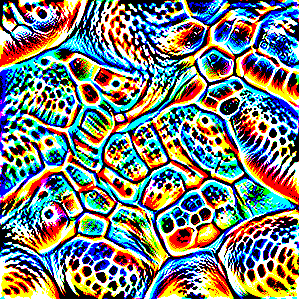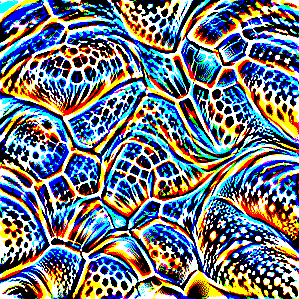leatherback turtle, leatherback, leathery turtle, Dermochelys coriacea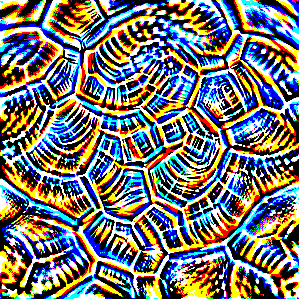mud turtle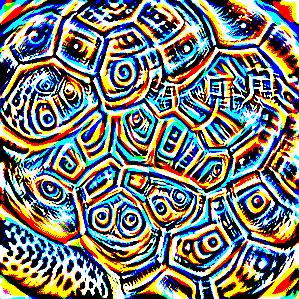terrapin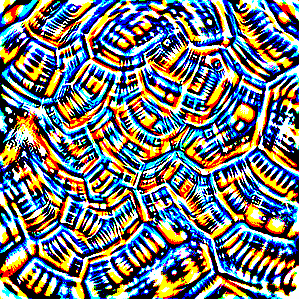box turtle, box tortoise

These images are vibrant, clear, interpretable, and obviously highly related to the target class. We can study these and see what the neural network has learned about each turtle class.

This is not just constrained to turtles, of course, but can be done with any imagenet class. I have created 1,000 such images, one for every class.

Large cats:leopard, Panthera pardus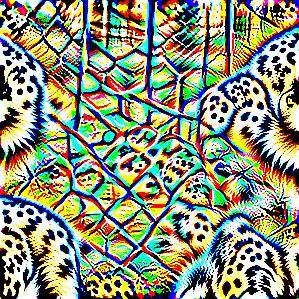snow leopard, ounce, Panthera uncia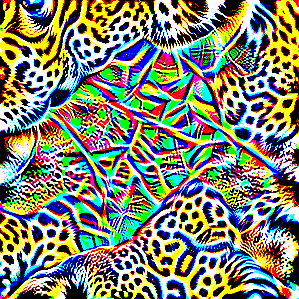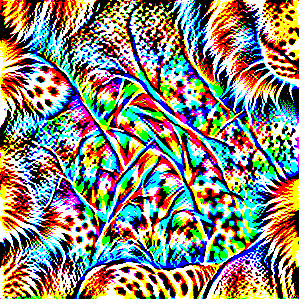lion, king of beasts, Panthera leo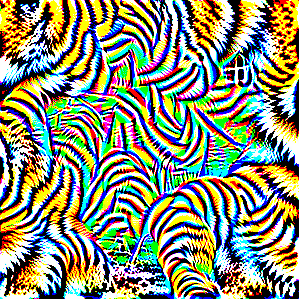tiger, Panthera tigris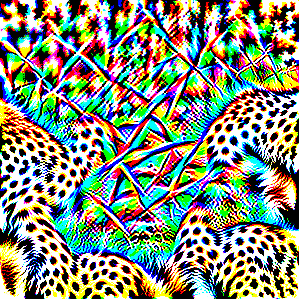cheetah, chetah, Acinonyx jubatus

# Diagnosis

Studying the images, it is possible to identify problems with the network, or perhaps with the dataset that it was trained on.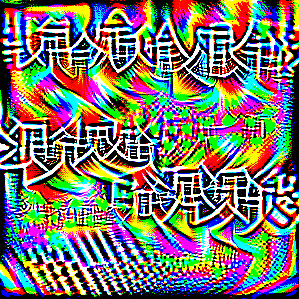monitor

Is that Chinese on the monitor? No it's not actual Chinese, but it is strange proto-Chinese that has been generated by the process. The network has learned that Chinese written over the image is a very strong indicator that it is a monitor. If you look at the imagenet training data, you will see that a large portion of the monitor images have been sourced from Alibaba and they have Chinese overlayed on the image.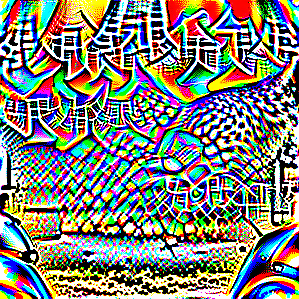motor scooter, scooter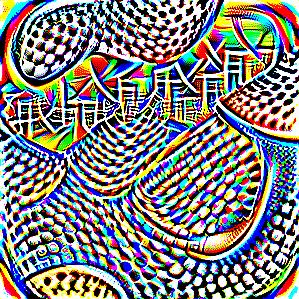sandal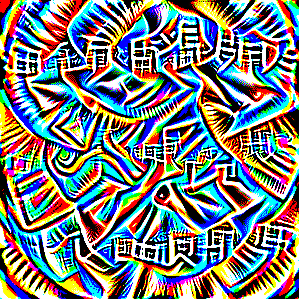shower cap

Monitor is not the only object with this problem, though it is perhaps the most striking.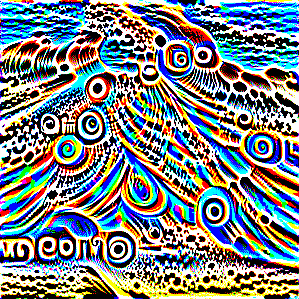seashore, coast, seacoast, sea-coast

Tourism is big business, and so a lot of the seashore images have English written on them, hence the funny proto-English above.toucan

There is a distinct cage or net visible for the toucan. From a quick glance at the training data, about half of toucan images include some cage or net. This is not something that we would want our network to learn if we were training it to identify toucans in the wild!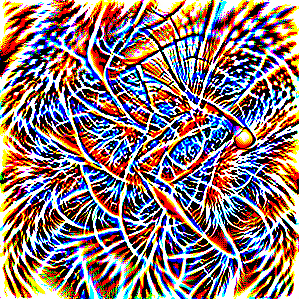tick

Clearly the network has learned a lot about where you find ticks, not just what they look like. Here is a tuck buried in some long hair.

# Creating Targeted Universal Images: The Math

Creating a targeted universal image looks a lot like the routine for training a neural network, but instead of training the network to make the correct output, we train an image to make the network output the target instead of what is correct.

We use the crossy entropy loss, as was used to train the network.

The goal is to maximize the expected output for the target, $$p_{target}$$, when the change $$\delta$$ is made to a randomly selected image $$x$$.

$$\max_{\delta}E_x[p_{target}]$$

We constrain the $$\delta$$ to be to be no more than some $$\epsilon$$ for each pixel value. This is the infinity norm constraint.

$$\lvert\lvert\delta\lvert\lvert_\infty \leq \epsilon$$

### Change of Variable

We perform a change of variable in order to do unconstrained optimization. For each pixel value $$i$$ we define a $$\delta_i$$ function that maps a new variable $$w_i \in [-\infty,\infty]$$ to $$\delta_i$$ values that obey the constraint:

$$\delta_i(w_i) = \epsilon\cdot tanh(w_i)$$

Since $$tanh(w) \in (-1,1)$$

then $$\delta_i(w_i) \in (-\epsilon,\epsilon)$$

We can them perform unconstrained stochastic gradient descent with optimizers like Adam.

### Optimization

Given a random $$x$$ from the dataset, we compute $$p_{target} = f_{target}(x + \delta(w))$$ where $$f$$ is the target neural network (i.e. inception v3).

Then we compute the gradient $$\frac{\partial p_{target}}{\partial w}$$, pass it to the Adam optimizer, and take a step to maximize $$p_{target}$$.

### Improving Results with Augmentations

Deep Neural Networks are very easily fooled. Unless we do something, our optimization routine is likely to converge on images that exploit this weakness, rather than utilize the true properties of the target class. You can see some of this in the images that I have created, though I have taken steps to mitigate it. For example, the center of the big cat images above are largely trained to exploit DNN weaknesses rather than look like big cats.

We insert differentiable image augmentations between the changed image and the target network. $$\delta$$ is calculated and added to the image. The result is clipped to be a valid image $$x + \delta \in [0,255]$$. The image is randomly flipped horizontally, blurred with a Gaussian kernel, and randomly cropped. Finally the image is bilinearly resized back up to (299,299) as expected by inception v3.

The augmetations can be thought of as a function $$A(X)$$ and we can consider our optimization to be seeking to maximize:

$$\max_{w}E_x[f_{target}(A(x + \delta(w)))]$$

# Conclusion

When I began working with adversarial images I felt that therin lay some essential truth behind the functioning of neural networks. What I demonstrate here is a technique that offers diagnostic power in assessing a DNN CNN image classifier.